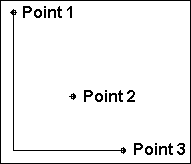# Ortho Line 2

Like the Ortho Line command, this command draws lines that are parallel to the X, Y, or Z axis. However, the points set in this command function differently.

Point 1: Beginning of the line

Point 2: Direction of 1st segment

Point 3: Length of 1st segment; length & direction of 2nd segment

Points 4-n: even number: direction of segment; odd number: previous segment length; current segment length & direction

With the Ortho Line-2 command, the first point set is the beginning of the line. The second point determines only the direction of the first line segment, not the length. After the second point is set, the length of the first line segment continues to change when the cursor moves. The third point determines the direction of the second line segment and the length of both the first and second line segments. After the third point is set, all even numbered points function like the second point, determining only the direction of the line segment. All the following odd numbered points work like the third point and determine the length of the previous line segment and the length and direction of the current line segment.

NOTE: This command only works in 2D Mode. Also, Orthogonal Mode needs to be disabled.

Example: Draw a bent line.

Choose the Ortho Line-2 command. Set a point for the beginning of the line. Now, move the cursor down and to the right. Notice how the rubber-band line will only move straight along the X-Axis or the Y-Axis. Set a point so that the line goes down along the Y-Axis. Now, move the cursor up and down. Notice how the length of the first segment gets longer or shorter with the movement of the cursor. Move the cursor to the right and set a point so that the line forms an “L” shape. Press Enter to complete the command and add the line to the drawing.